# Graphs, Properties & the Concept of Change

Instructor: Matthew Bergstresser

Matthew has a Master of Arts degree in Physics Education. He has taught high school chemistry and physics for 14 years.

Graphs are used in many different fields. They are used to show the relationship between variables. In this lesson, we will investigate how graphs can visually represent changes. Updated: 12/14/2020

## Change

You may have heard the old quote, ''There is nothing permanent except for change.'' Time marches on and can't be stopped. With time changes, come all sorts of other changes. Graphs are very useful to plot data and analyze what is changing. Let's look at the properties of graphs.

## Properties of Graphs

A common graph that is useful for plotting data has two axes. The y-axis is usually vertical, and the x-axis is typically horizontal.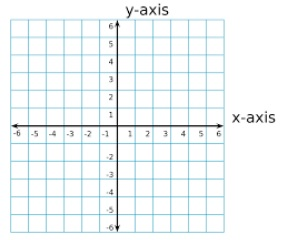Both axes need to be labeled to indicate what they represent, and maximum values should be determined based on the data set to be plotted. The location where the x and y axes intersect is called the origin. This gives the grid four quadrants. The top right is Quadrant 1, the top left is Quadrant 2, the bottom left is Quadrant 3 and the bottom right is Quadrant 4.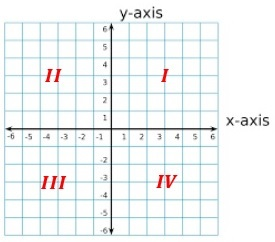The x-axis contains the domain, and the y-axis contains the range. The domain is also known as the independent variable because changes in it are not affected by the other variable. For example, when time is involved in a scenario it is an independent variable because time isn't affected by anything else. The range is known as the dependent variable because changes in it are dependent on the independent variable. For example, outdoor air temperatures are affected by the time of day, therefore temperature is a dependent variable.

### Plotting Points

Before we can determine the rate of change of a graph we need to plot a data set. Data sets are always written between parenthesis with the x-value listed first followed by a comma and then the y-value. For example, in Graph 1, the blue and red lines intersect at the point (2,3), which is in Quadrant I. Any point in Quadrant I has positive x and y-values. Quadrant II has negative x-values, and positive y-values. Quadrant III has negative x and y-values, and Quadrant IV has positive x-values and negative y-values.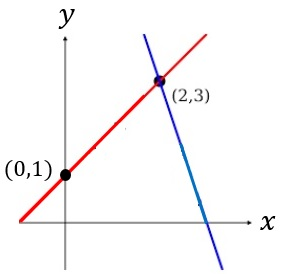### Slope

Slope is the rate of change in the data plotted on the grid. It can be calculated using the slope formula.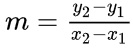• m is the slope
• x1 and y1 are the domain and range of any point on the line.
• x2 and y2 are the domain and range of any other point on the line.

Let's look back at Graph 1, and determine the slopes of the red line.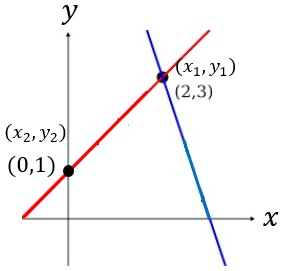Let's plug in the two points into the slope formula.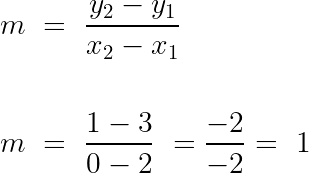Since this graph doesn't have labels on the x and y-axes, this slope doesn't have any meaning other than y changes 1.0 units for every 1 x-unit. Let's look at an example where slope has physical meaning.

## Rainfall During Tropical Storm

Tropical storms drop lots of rain. Graph 2 shows the amount of rainfall per hour from Tropical Storm Gunther.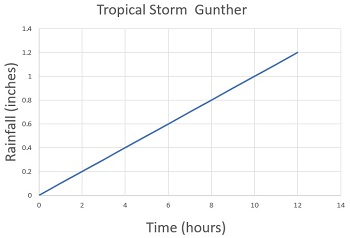To unlock this lesson you must be a Study.com Member.

### Register to view this lesson

Are you a student or a teacher?